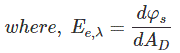φs is the received radiant flux on the detector surface and AD is the detector area or surface.
Irradiance always follows the Inverse Square Law. Suppose from a point source the radiant flux is being received by two surfaces of A1 and A2 where they are equal surface area. They are placed at r1 and r2 distance.Now the flux received by the surface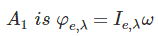And the flux received by the surfaceWhere, Ie,λ radiant intensity and ω solid angle.

Again the radiant flux received per unit area for A1 and A2 are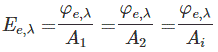Here A1 and A2 are equal.
Putting the φe,λ = Ie,λ ω in the equation we get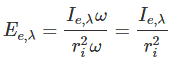This is Inverse Square Law of irradiance.

If we convert this irradiance into Illuminance then we should follow the conversion equation i.e.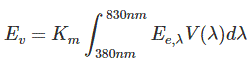Where, Km is the constant which is called maximum spectral luminous efficacy and its value is 683 lm/W.
By definition the luminous flux received by unit area of the detector is called Illuminance.
Its unit is Lux or Lumen per sq. meter (lm/sq. m).
It also follows the same inverse square law, i.e.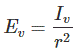Ev is related to the surface dA where luminous flux is falling on this surface perpendicularly.
E’v is related to the surface dA’ where this surface creates an angle Ɵ to the base plane.
As per figure above,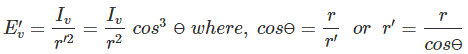This above equation can be written making it generalized,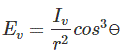Want To Learn Faster? 🎓
Get electrical articles delivered to your inbox every week.
No credit card required—it’s 100% free.